# CBSE Physics for Class 12 | Electrostatics | Methods of Charging.

## Methods of Charging.

A body can be charged by following methods :

### (1) Charging By friction :

In friction when two bodies are rubbed together, electrons are transferred from one body to the other.

As a result of this one body becomes positively charged while the other negatively charged, e.g., when a glass rod is rubbed with silk, the rod becomes positively
charged while the silk negatively.

However, ebonite on rubbing with wool becomes negatively charged making the wool positively charged.

Clouds also become charged by friction.

In charging by friction in accordance with conservation of charge, both positive and negative charges in equal amounts appear simultaneously due to transfer of electrons from one body to the other.

### (2) Charging By electrostatic induction :

If a charged body is brought near an uncharged body, the charged body will attract opposite charge and repel similar charge present in the uncharged body.

As a result of this one side of neutral body (closer to charged body) becomes oppositely charged while the other is similarly charged.

This process is called electrostatic induction.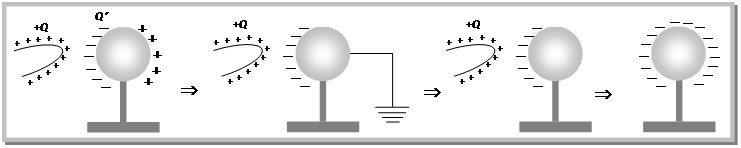Inducting body neither gains nor loses charge.

Induced charge can be lesser or equal to inducing charge (but never greater) and its maximum value is given by$\displaystyle Q'=-Q\left[ 1-\frac{1}{K} \right]$ where Q is the inducing charge and K is the dielectric constant of the material of the uncharged body. Dielectric constant of different media are shown below

 Medium K Vacuum / air Water Mica Glass Metal 1 80 6 5–10 ¥

Dielectric constant of an insulator can not be$\displaystyle \infty$

For metals in electrostatics$\displaystyle K=\infty$ and so$\displaystyle Q'=-\,Q;$ i.e. in metals induced charge is equal and opposite to inducing charge.

### (3) Charging by conduction :

Take two conductors, one charged and other uncharged. Bring the conductors in contact with each other.

The charge (whether$\displaystyle -ve$or$\displaystyle +ve$) under its own repulsion will spread over both the conductors. Thus the conductors will be charged with the same sign.

This is called as charging by conduction (through contact).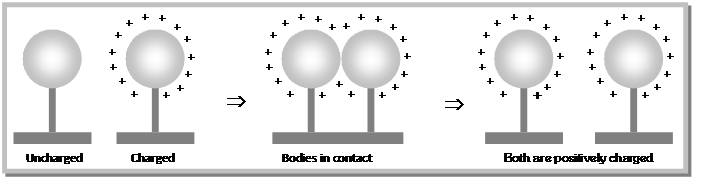## Electroscope.

It is a simple apparatus with which the presence of electric charge on a body is detected (see figure).

When metal knob is touched with a charged body, some charge is transferred to the gold leaves, which then diverges due to repulsion.

The separation gives a rough idea of the amount of charge on the body.

If a charged body brought near a charged electroscope the leaves will further diverge.

If the charge on body is similar to that on electroscope and will usually converge if opposite.

If the induction effect is strong enough leaves after converging may again diverge.

### (1) Uncharged electroscope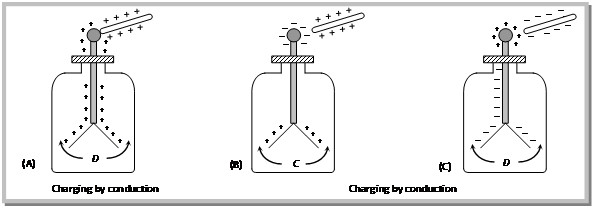### (2) Charged electroscope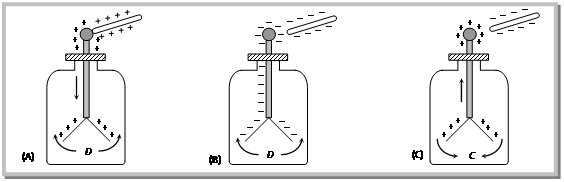# CBSE Physics for Class 12 | Electrostatics | Electric Charge

## Electric Charge.

### Definition of Electric Charge :

Charge is the property associated with matter due to which it produces and experiences electrical and magnetic effects.

### Origin of electric charge :

It is known that every atom is electrically neutral, containing as many electrons as the number of protons in the nucleus.

Charged particles can be created by disturbing neutrality of an atom.

Loss of electrons gives positive charge (as then np> ne) and gain of electrons gives negative charge (as then ne > np) to a particle.

When an object is negatively charged it gains electrons and therefore its mass increases negligibly.

Similarly, on charging a body with positive electricity its mass decreases.

Change in mass of object is equal to ´ me.

Where, n is the number of electrons transferred and$\displaystyle {{m}_{e}}$ is the mass of electron$\displaystyle =9.1\times {{10}^{-31}}Kg$.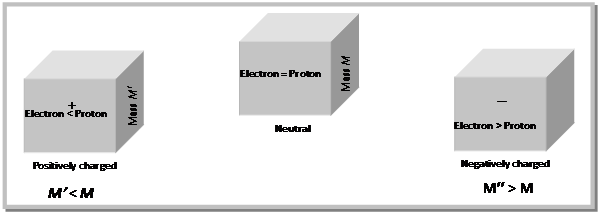### Type of Electric Charge:

There exists two types of charges in nature

1. Positive charge
2. Negative charge

Charges with the same electrical sign repel each other, and charges with opposite electrical sign attract each other.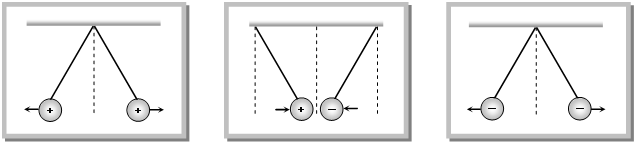### Unit and dimensional formula of Electric Charge:

Rate of flow of electric charge is called electric current i.e.,$\displaystyle i=\frac{dQ}{dt}$ Þ$\displaystyle dQ=idt$, hence S.I. unit of charge is – Ampere ´ sec = coulomb (C), smaller S.I. units are mC, mC, nC$\displaystyle (1mC={{10}^{-3}}C,\,1\mu \,C={{10}^{-6}}C,\,1nC={{10}^{-9}}C)$. C.G.S. unit of charge is – Stat coulomb or e.s.u.

Electromagnetic unit of charge is – ab coulomb$\displaystyle 1C=3\times {{10}^{9}}\,stat\,coulomb=\frac{1}{10}\,ab\,coulomb$.

Dimensional formula of electric charge$\displaystyle [Q]=\left[ AT \right]$

Benjamin Franklin was the first to assign positive and negative sign of charge.

The existence of two type of charges was discovered by Dufog.

Franklin (i.e., e.s.u. of charge) is the smallest unit of charge while faraday is largest (1 Faraday = 96500 C).

The e.s.u. of charge is also called stat coulomb or Franklin (Fr) and is related to e.m.u. of charge through the relation$\displaystyle \frac{\text{emu of charge}}{\text{esu of charge}}=3\times {{10}^{10}}$

### Point charge :

A finite size body may behave like a point charge if it produces an inverse square electric field.

For example an isolated charged sphere behave like a point charge at very large distance as well as very small distance close to it’s surface.

### Properties of Electric charge

(i) Electric Charge is transferable : If a charged body is put in contact with an uncharged body, uncharged body becomes charged due to transfer of electrons from one body to the other.

(ii) Electric Charge is always associated with mass, i.e., charge can not exist without mass though mass can exist without charge.

(iii) Electric Charge is conserved : Charge can neither be created nor be destroyed. e.g.
In radioactive decay the uranium nucleus (charge$\displaystyle =+\,92e$) is converted into a thorium nucleus (charge$\displaystyle =+90e$) and emits an$\displaystyle \alpha$-particle (charge$\displaystyle =+2e$)$\displaystyle _{92}{{U}^{238}}{{\to }_{90}}T{{h}^{234}}{{+}_{2}}H{{e}^{4}}$. Thus the total charge is$\displaystyle +92e$ both before and after the decay.

(iv) Invariance of Electric charge : The numerical value of an elementary charge is independent of velocity.

It is proved by the fact that an atom is neutral.

The difference in masses on an electron and a proton suggests that electrons move much faster in an atom than protons.

If the charges were dependent on velocity, the neutrality of atoms would be violated.

(v) Electric Charge produces electric field and magnetic field : A charged particle at rest produces only electric field in the space surrounding it.

However, if the charged particle is in unaccelerated motion it produces both electric and magnetic fields.

And if the motion of charged particle is accelerated it not only produces electric and magnetic fields but also radiates energy in the space surrounding the charge in the form of electromagnetic waves.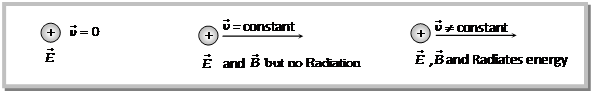(vi) Electric Charge resides on the surface of conductor : Charge resides on the outer surface of a conductor because like charges repel and try to get as far away as possible from one another and stay at the farthest distance from each other which is outer surface of the conductor.

This is why a solid and hollow conducting sphere of same outer radius will hold maximum equal charge and a soap bubble expands on charging.

(vii) Electric Charge leaks from sharp points : In case of conducting body no doubt charge resides on its outer surface, if surface is uniform the charge distributes uniformly on the surface and for irregular surface the distribution of charge, i.e., charge density is not uniform.

It is maximum where the radius of curvature is minimum and vice versa. i.e.,$\displaystyle \sigma \propto$$\displaystyle \left( 1/R \right)$. This is why charge leaks from sharp points.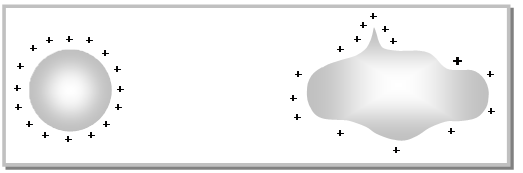(viii) Quantization of Electric charge : When a physical quantity can have only discrete values rather than any value, the quantity is said to be quantised. The smallest charge that can exist in nature is the charge of an electron. If the charge of an electron ($\displaystyle =1.6\times {{10}^{-19}}C$) is taken as elementary unit i.e. quanta of charge the charge on any body will be some integral multiple of e
i.e.,$\displaystyle Q=\pm \,\,ne$ with$\displaystyle n=1,\,2,\,3\,....$

Charge on a body can never be$\displaystyle \pm \frac{2}{3}e$,$\displaystyle \pm 17.2e$ or$\displaystyle \pm {{10}^{-5}}e$etc.

Note :

• Recently it has been discovered that elementary particles such as proton or neutron are composed of quarks having charge$\displaystyle \left( \pm 1/3 \right)$e and$\displaystyle \left( \pm 2/3 \right)$e. However, as quarks do not exist in free state, the quanta of charge is still e.
• Quantization of charge implies that there is a maximum permissible magnitude of charge.

Comparison of Charge and Mass.

We are familiar with role of mass in gravitation, and we have just studied some features of electric charge. We can compare the two as shown below

 Charge Mass (1) Electric charge can be positive, negative or zero. (1) Mass of a body is a positive quantity. (2) Charge carried by a body does not depend upon velocity of the body. (2) Mass of a body increases with its velocity as$\displaystyle m=\frac{m{}_{0}}{\sqrt{1-{{v}^{2}}/{{c}^{2}}}}$ where c is velocity of light in vacuum, m is the mass of the body moving with velocity v and$\displaystyle m{}_{0}$ is rest mass of the body. (3) Charge is quantized. (3) The quantization of mass is yet to be established. (4) Electric charge is always conserved. (4) Mass is not conserved as it can be changed into energy and vice-versa. (5) Force between charges can be attractive or repulsive, according as charges are unlike or like charges. (5) The gravitational force between two masses is always attractive.